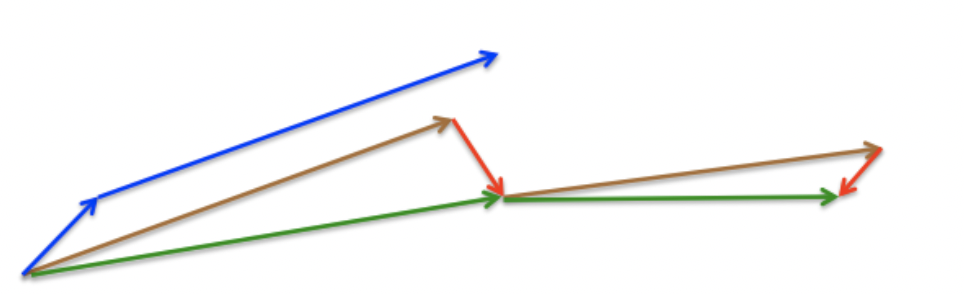# NAG¶

SGD 还有一个问题是困在局部最优的沟壑里面震荡。想象一下你走到一个盆地，四周都是略高的小山，你觉得没有下坡的方向，那就只能待在这里了。可是如果你爬上高地，就会发现外面的世界还很广阔。因此，我们不能停留在当前位置去观察未来的方向，而要向前一步、多看一步、看远一些。

NAG全称Nesterov Accelerated Gradient，是在SGD、SGD-M的基础上的进一步改进，我们知道在时刻$$t$$的主要下降方向是由累积动量决定的，自己的梯度方向说了也不算，那与其看当前梯度方向，不如先看看如果跟着累积动量走了一步，那个时候再怎么走。因此，NAG不计算当前位置的梯度方向，而是计算如果按照累积动量走了一步，那个时候的下降方向：

$v_{t}=\gamma v_{t-1}+\eta \nabla_{\theta}J(\theta-\gamma v_{t-1})$

NAG参数更新公式如下，其中$$\eta$$是学习率， $$\nabla_{\theta}J(\theta-\gamma v_{t-1})$$是当前参数的梯度

$\theta=\theta-v_{t}$• 有利于跳出当前局部最优的沟壑，寻找新的最优值，但收敛速度慢